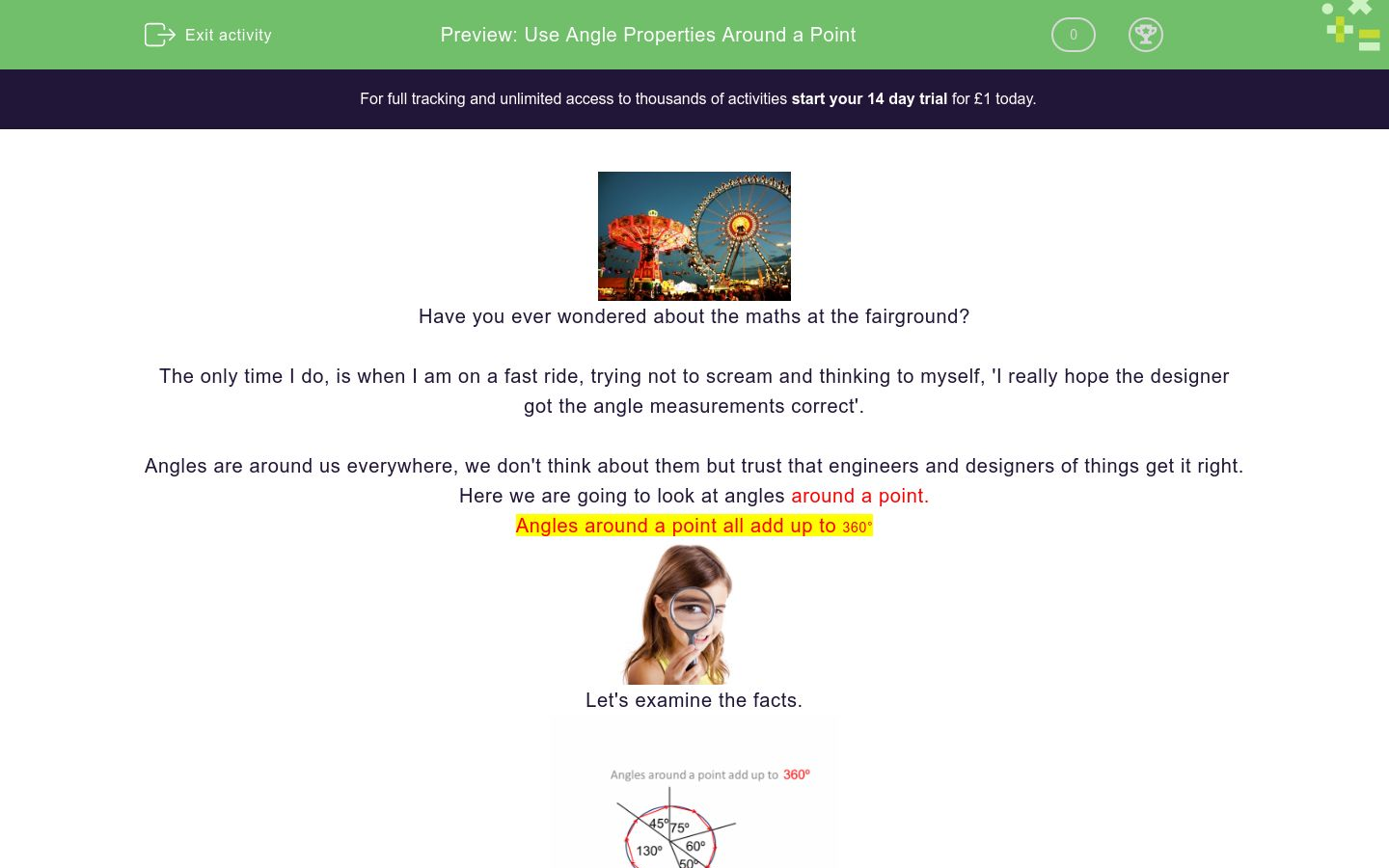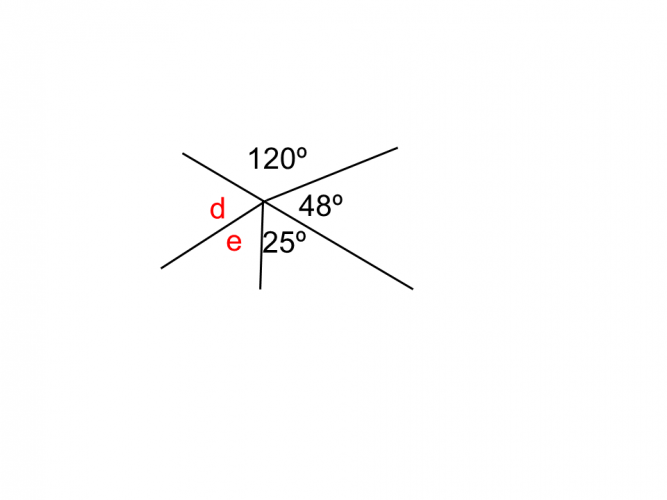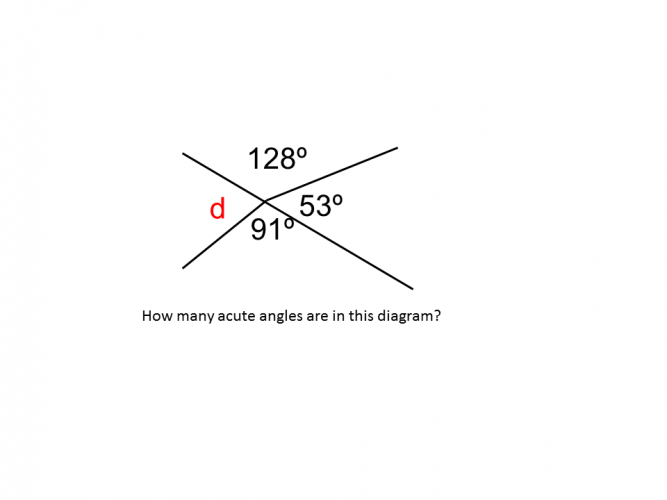# Use Angle Properties Around a Point

In this worksheet, students will learn about angle properties around a point. They will also be able to find missing angles using number and algebra.Key stage:  KS 4

GCSE Subjects:   Maths

GCSE Boards:   AQA, Eduqas, OCR, Pearson Edexcel

Curriculum topic:   Geometry and Measures, Basic Geometry

Curriculum subtopic:   Properties and Constructions, Angles

Difficulty level:### QUESTION 1 of 10Have you ever wondered about the maths at the fairground?

The only time I do, is when I am on a fast ride, trying not to scream and thinking to myself, 'I really hope the designer got the angle measurements correct'.

Angles are around us everywhere, we don't think about them but trust that engineers and designers of things get it right.

Here we are going to look at angles around a point.

Angles around a point all add up to 360°Let's examine the facts.Adding up all the angles should come to 360°.  If they don't sack the designer.

To find a missing angleAdd up the angles that you are given 105° + 110° + 48° = 263°

Now subtract from 360°

360° - 263° = 97°

Let's take our new found skills for a spin.Calculate the value for angle C.283°

177°

77°

83°Find the missing angle193° 167° 83.5° 105.5° angle d angle eThere are one, two, three acute angles in this diagram.What does each angle at the centre of this ball measure?

30°

40°

50°

60°150°

160°

170°

180°Find the value of x.130° 120° 94° 24° 32° 72° 98° x is equal to 3x is equal to 5x is equal toThis clock face is divided into 5 minute segments.

What is the angle of one segment at the centre of the clock?

 130° 120° 94° 24° 32° 72° 98° x is equal to 3x is equal to 5x is equal to
• Question 1EDDIE SAYS
Was your head in a spin, or were you spinning with success? Either way there is lots more practice to come. 117 + 48 + 79 = 244° 360 - 244 = 116°
• Question 2Calculate the value for angle C.77°
EDDIE SAYS
Did you know snowboarders use the term 360 for one of their moves? When they fall over, you can calculate by how much they missed the landing. I would let them regain their pride first though. Here the snowboarder made it only so far. 132 + 63 + 88 = 283° 360 - 283 = 77°
• Question 3Find the missing angle140
EDDIE SAYS
Did you know the 360 is a famous model of electric guitar made by the Rickenbacker corporation? Is that why some musicians swing their arms a full 360 when in concert? This guitarist missed it by a little. 90 + 25 + 105 = 220° 360 - 220 = 140°
• Question 4193° 167° 83.5° 105.5° angle d angle e
EDDIE SAYS
Did you know that it was decided that there were 360° in a circle by the Egyptians.? They discovered this by studying the earth moving around the sun in a year. Back then they worked on a 360 day year. Let's move around this point to find the missing angles. 120 + 48 + 25 = 193° 360 - 193 = 167° We are not told anything to the contrary so we have to assume the two angles are both the same size. 167 ÷ 2 = 83.5° Clever people the Egyptians.
• Question 5There are one, two, three acute angles in this diagram.
EDDIE SAYS
This is just a variation on a theme. You need to recall the fact that acute angles are less than 90° We can see that there is one in the diagram already, we just have to work out the missing angle to see if there are two. 128 + 53 + 91 = 272° 360 - 272 = 88° So there are two. Why was the obtuse angle upset? Because he wasn't a cute.
• Question 6What does each angle at the centre of this ball measure?

60°
EDDIE SAYS
I hope this didn't throw you off. You have all the information you need. There are six equal angles at the centre of the ball so just take 360 and divide by six. 360 ÷ 6 = 60°
• Question 7150°
EDDIE SAYS
I hope this didn't get your head into two much of a spin. it is not as bad as it looks..honest. Use your knowledge. The ratio totals add up to 12. Divide 360 by 12 360 ÷ 12 = 30 The largest ratio is 5 parts 30 x 5 = 150° Now when your maths teacher asks yo if you understand angles, you can say 'yes to a degree'.
• Question 8Find the value of x.

32.8
EDDIE SAYS
Good old algebra, it just can't keep its nose out of anything. It likes to get straight to the point. 105 + 91 = 196° 360 - 196 = 164° 164 is the value of 5 lots of x. To find x 164 ÷ 5 = 32.8°
• Question 9130° 120° 94° 24° 32° 72° 98° x is equal to 3x is equal to 5x is equal to
EDDIE SAYS
As long as you remember the 360 rule then this shouldn't take you around the houses to much. There are 15 lots of x altogether. To find the value of x take 360 and divide by 15. 360 ÷ 15 = 24° Now multiply up to find the others. 3 x 24 = 72° 5 x 24 = 120°
• Question 10This clock face is divided into 5 minute segments.

What is the angle of one segment at the centre of the clock?

EDDIE SAYS
Let's take our time to think about this. Count the number of segments the clock has been divided into. There are 12. We know there are 360° in any circle. 360 ÷ 12 = 30°
---- OR ----

Sign up for a £1 trial so you can track and measure your child's progress on this activity.

### What is EdPlace?

We're your National Curriculum aligned online education content provider helping each child succeed in English, maths and science from year 1 to GCSE. With an EdPlace account you’ll be able to track and measure progress, helping each child achieve their best. We build confidence and attainment by personalising each child’s learning at a level that suits them.

Get started